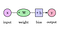# Cat or Dog?

We can see this concept in a boy named Timmy. Timmy was learning how to tell the difference between a cat and a dog. Timmy has never seen any of these things before or even knows what they are, but after a while, he was able to detect the difference between a cat and a dog. Here’s the thing, Timmy isn’t a real person. Timmy is just a machine that was programmed to learn how to tell the difference between a cat and a dog. So, how could this machine learn how to tell the difference. Timmy learned through the use of Machine Learning.

# Machine Learning … What’s That?

As humans, we learn in many different ways. Some people learn on their own, some learn just enough so that they can learn the rest on their own, but how can we make a machine do that. This is where Supervised, Unsupervised, and Semi-Supervised Learning come in to play.

## Supervised Learning

This is how the machine trains, but Well, for starters this is a method used to train a machine. What this means is that, when you give data to the machine with the answers. At first, we would give Timmy a picture of a dog, and label it as “Dog”. We would do the same thing with cats and label it with “Cat”. Let’s say we show Timmy 50 images of dogs labelled with “Dogs”, and 50 images of cats labelled with “Cats”. After the machine would now be able to tell the difference between a cat and a dog, so if we show a picture of a cat, it would know that it's a cat.

## Unsupervised

Unsupervised Learning is the opposite of Supervised Learning. We would just let the machine run and do whatever it wants. How is it going to learn? We would use something called a rewarding system. This is where if a machine does what you want it to do, or close to what you would want to do, you would give the machine a point. If they do the opposite you would lose a point. The goal of the machine is to get the most amount of points as possible. Since the machine is doing random actions, if it loses a point, it would have a lower chance of doing that action, and vice-versa with gaining a point. Once you keep letting the machine do this, it will slowly start to learn and realize what it needs to do. Pretty cool right?

# Let’s Dive Deeper into Machine Learning

To properly understand what a Neural network is in Deep Learning, let’s look back at our example with Timmy the Robot. When we give Timmy an image of a dog, that would be the input. The answer which could either be dog or cat is going to be the output. So Timmy will have to look at the picture, and analyze every detail of the image. Depending on what a machine analyzes, it will change the values of each hidden layer. The hidden layers are what is in between the input and output layers. These layers can vary depending on what you want the machine to learn/do. Each part of the input layer is connected to each part of the first hidden layer, and each part of the last hidden layer is connected to each part of the output layer, and all the hidden layers between are connected. They are all connected by synapses which change to the value of each layer depending on the previous one.

## Weights & BiasesFigure 6: Shows how weights and biases are used to find the output

As I mentioned before, when we give input to a machine it changes the value of each layer depending on the previous input. This is basically what weight is, it decides how much influence the input would have on the output. Now, what are biases? Biases will always add input to the next layer that always has a value of 1. In turn, weights are what decides the changed value, whereas biases add value to any input with the value of one.

# What Did We Talk About?

## We talked about Machine Learning:

• Supervised, Unsupervised, and Semi-Supervised Learning
• Deep Learning/ Neural Networks
• Gave an example of how Machine Learning is being used.